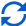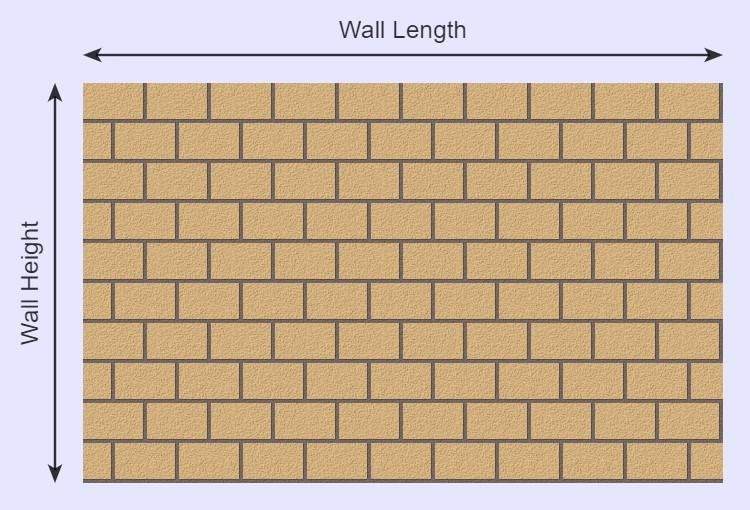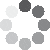Enter parameters
Enter parameters
Wall Length:
ft
• inches (in)
• feet (ft)
• yards (yd)
• centimeters (cm)
• meters (m)
Wall Height:
ft
• inches (in)
• feet (ft)
• yards (yd)
• centimeters (cm)
• meters (m)
Brick Length:
in
• inches (in)
• feet (ft)
• yards (yd)
• centimeters (cm)
• meters (m)
Brick Height:
in
• inches (in)
• feet (ft)
• yards (yd)
• centimeters (cm)
• meters (m)
Mortar joint:
in
• inches (in)
• feet (ft)
• yards (yd)
• centimeters (cm)
• meters (m)
Price Per Brick:
Waste (%):Result
Wall Area:
0
ft²
• square feet (ft²)
• square inches (in²)
• square yards (yd²)
• square miles (mi²)
• acres (ac)
• square centimeters (cm²)
• square meters ()
Bricks:
0
Cost:
$(2 votes, average: 5.00 out of 5)Loading... Similar Calculators: Real-time graphics. Make the calculations and see the changes. Embed Similar Calculators:When starting any outdoor landscaping or construction project, having an idea of how much material you will need is important before you get started. You know that buying the correct number of bricks when building a wall will save you both time and money. Our brick calculator can help you do this. 😉 With a little preparation, it can ensure that your next construction project will get off to an efficient start. In this article we will detail how to use the calculator and how it works. Contents: ## Brick calculator formulaBased on the measurements you enter, the brick calculator first works out the total area of the wall: $$Wall\,Area = Wall\,Length \times Wall\,Height$$ and the area of each brick: $$Brick\,Area = Brick\,Length \times Brick\,Height$$ ### Calculating the Total Number of Bricks To calculate the total number of bricks required in the wall, you will need to know the distance between the bricks occupied by the mortar (the mortar joint) and how much wastage of bricks occurs (in percent). $$Number\,of\,bricks= {Wall\,Area \over (Brick\,Length + Mortar\,Joint) \times (Brick\,Height + Mortar\,Joint)} + Waste\,(\%)$$ ### How to Add Waste Percent? Let’s imagine we needed 100 bricks, but want to account for 12% waste (allowing for bricks breaking, etc.). We would calculate 12% of 100, which is 12, and add this value to the 100 bricks required. I would therefore require 112 bricks to account for this waste. NB: Brick wastage is commonly between 5% for hard bricks and up to 15% for softer ones. ### Calculating Total Cost of the Building Materials Finally, if you know the price per brick the calculator can work out the total cost of the bricks using the formula: $$Cost = Number\,of\,Bricks \times Price\,Per\,Brick$$ If you don’t know the unit price of a brick, you can easily calculate it using the formula: $$Price\,Per\,Brick = {Total\,Cost\,of\,Bricks \over Number\,of\,Bricks}$$ Confused? 😵 See below for a full example, detailing how to calculate the number of bricks, price per brick and total cost of the bricks. Otherwise, enter your measurements, estimates and dimensions into the online calculator to get your next construction project off to an efficient start! ## Example calculations Let’s say that I want to build a wall that measures 5 feet in length and 4 feet in width. For a building material, I will use bricks measuring 8 inches by 3 inches separated with mortar 0.3 inches wide and assume a brick wastage of 5%. 🧱 Entering these dimensions into the calculator gives a wall area (in square feet) of: $$Wall\,Area = Wall\,Length \times Wall\,Height = 5\,ft \times 4\,ft = 20\,ft^2$$ and a brick area (in square inches) of: $$Brick\,Area = Brick\,Length \times Brick\,Height = 8\,in \times 3\,in = 24\,in^2$$ The total number of bricks required is given by the following calculations: $$Number\,of\,bricks = {Wall\,Area \over (Brick\,Length + Mortar\,Joint) \times (Brick\,Height + Mortar\,Joint) } + Waste\,(\%) = {20\,ft^2 \over (8\,in + 0.3\,in) \times (3\,in + 0.3\,in)} + 5\,(\%) = 111$$ Imagine I don’t know how much an individual brick costs, but I can purchase 500 bricks at a cost of$600. I can therefore calculate the price per brick:

$$Price\,Per\,Brick = {Total\,Cost\,of\,Bricks \over Number\,of\,Bricks} = {600 \over 500} = 1.2\,per\,brick$$

The calculator can therefore estimate the total cost of the building material:

$$Cost = Number\,of\,Bricks \times Price\,Per\,Brick = 111 \times 1.2 = 133.2$$

## What if my measurements are in other units?

Well, the answer couldn’t be easier! In the calculator there are many options for each unit of measurement.

Simply select the desired unit and our calculator will do the following conversions for you:

$$1\,foot = 12\,inches = 0.33\,yards = 30.48\,centimeters = 0.3048\,meters$$

It’s that simple! 🙂

Embed this calculator on your site!Add live graphics
Copied to clipboard! PreviewWhat's wrong?
Minimum 5 characters!